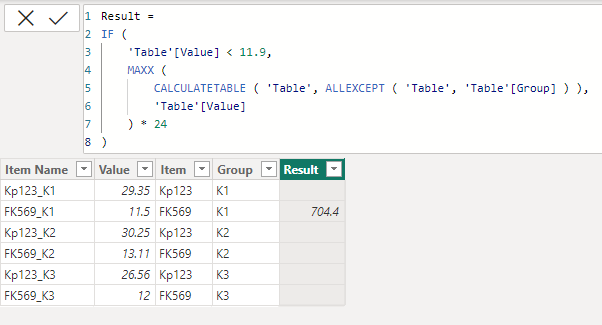cancel
Showing results for
Did you mean:Frequent Visitor

## Creating custom column with Multiple condition on Column

Hi All,

I have a table containing the list of multiple items and values like below

Item Name    Value
Kp123_K1      29.35
FK569_K1       11.5
Kp123_K2      30.25
FK569_K2      13.11
Kp123_K3       26.56
FK569_K3       12.00

In the new column, I should get if (item name(FK569_K1)<11.9) then item name(Kp123_K1 )* 24
if the condition is satisfied value will return( Ex.  29.35* 24) else false should be return.

Can any one help me the dax formula for this to get new custom column

Thank you for the help

1 ACCEPTED SOLUTIONCommunity Support

Hi @Lakshmi1294 ,

Here are the steps you can follow：

1. Create calculated column.

``````All1 =
var _if=
IF('Table'[Value]<11.9,1,0)
return
IF(
_if =0 ,'Table'[Value] *24)``````
``````Max1 =
IF('Table'[Value]<11.9
,
MAXX(ALL('Table'),[Value]) * 24,BLANK())``````

2. Result:Best Regards,

Liu Yang

If this post helps, then please consider Accept it as the solution to help the other members find it more quickly

3 REPLIES 3Community Support

Hi @Lakshmi1294 ,

Here are the steps you can follow：

1. Create calculated column.

``````All1 =
var _if=
IF('Table'[Value]<11.9,1,0)
return
IF(
_if =0 ,'Table'[Value] *24)``````
``````Max1 =
IF('Table'[Value]<11.9
,
MAXX(ALL('Table'),[Value]) * 24,BLANK())``````

2. Result:Best Regards,

Liu Yang

If this post helps, then please consider Accept it as the solution to help the other members find it more quicklySuper User

Hi @Lakshmi1294
No sure if I correctly understood the requirement. However, please try``````Result =
IF (
'Table'[Value] < 11.9,
MAXX (
CALCULATETABLE ( 'Table', ALLEXCEPT ( 'Table', 'Table'[Group] ) ),
'Table'[Value]
) * 24
)``````Frequent Visitor

Hi

I don't want to create group field, could you please tell me without out group and also  if value<11.9 it should pick up the above value of respective item values